Courses

# Test: BJT Characteristics & Biasing

## 10 Questions MCQ Test Topicwise Question Bank for Electronics Engineering | Test: BJT Characteristics & Biasing

Description
This mock test of Test: BJT Characteristics & Biasing for Electrical Engineering (EE) helps you for every Electrical Engineering (EE) entrance exam. This contains 10 Multiple Choice Questions for Electrical Engineering (EE) Test: BJT Characteristics & Biasing (mcq) to study with solutions a complete question bank. The solved questions answers in this Test: BJT Characteristics & Biasing quiz give you a good mix of easy questions and tough questions. Electrical Engineering (EE) students definitely take this Test: BJT Characteristics & Biasing exercise for a better result in the exam. You can find other Test: BJT Characteristics & Biasing extra questions, long questions & short questions for Electrical Engineering (EE) on EduRev as well by searching above.
QUESTION: 1

### In a transitor, the value of α is 0.97. The value of current gain of common emitter configuration is

Solution:

Current gain of CE configuration is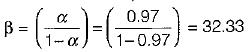QUESTION: 2

### A transistor has IB = 100 μA and IC = 2 mA. If IB changes by 25 μA and IC changes by 0.6 mA, the Changes in the value of β Would be

Solution: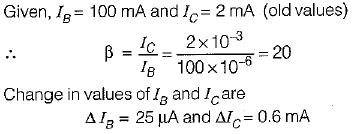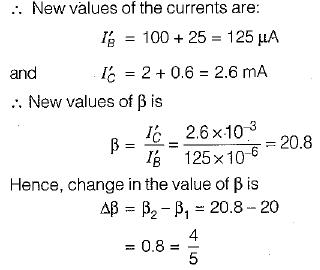QUESTION: 3

### For a transistor having α = 0.98, ICBO  = 5 μA and IB = 100 μA. The value of IC is

Solution: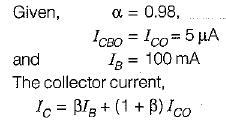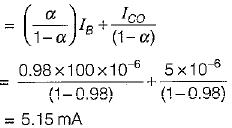QUESTION: 4

For the circuit shown below, the collector to emitter voltage is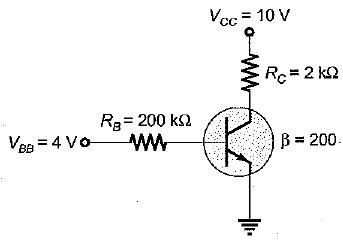Solution:

Let the transistor be operating under active region.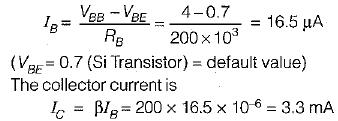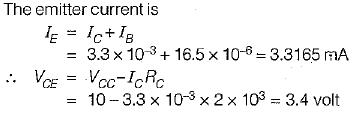QUESTION: 5

The reverse leakage current of the transistor when connected in CB configuration is 0.2 μA and it is 18 μA when the same transistor is connected in CE configuration. The value of α and β of the transistor for a base current of 30 mA will be respectively

Solution: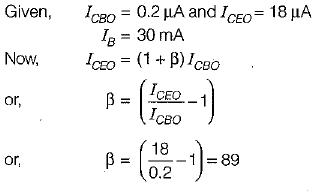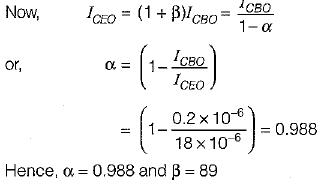QUESTION: 6

The value of Vc for the transistor shown in figure below is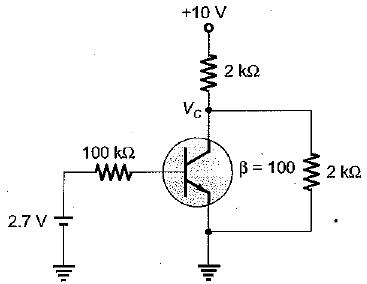Solution: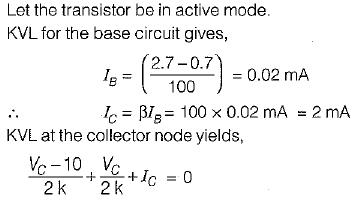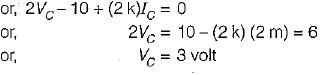QUESTION: 7

Match List-I (Transistor configuration) with List-ll (Applications) and select the correct answer using the codes given below the lists:
List-I
A. Common emitter
B. Common base
C. Common collector

List-ll
1. For impedance matching
2. For high frequency circuits
3. For audio frequency circuits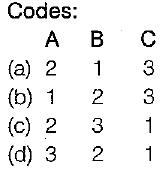Solution:
QUESTION: 8

Assertion (A): the dependency of base width on collector to emitter voltage is known as the “Early effect”.
Reason (R): Due to “Early effect”, α of the .transistor is increased by a larger value while maintaining a constant value of β.

Solution: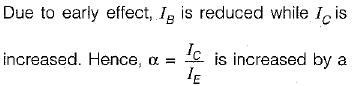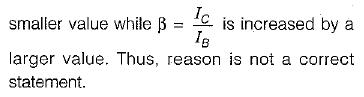QUESTION: 9

In forward mode n-p-n BJT, if we increase the voltage Vcc, the collector current increases

Solution:
QUESTION: 10

An emitter follower has high input impendance because

Solution: